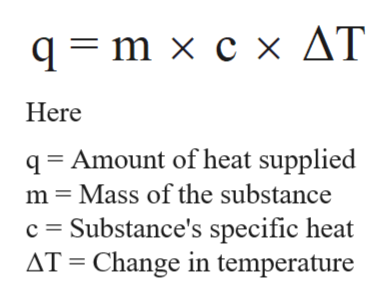12.755 g of a mental initially at 99.0 degrees C are placed into 75.0 g of water initially at 23 degrees C. The final temperature of the water is 24.5 degrees C. Calculate the specific heat of the metal. The specific heat of water is 4.184J/g degrees C

Question

12.755 g of a mental initially at 99.0 degrees C are placed into 75.0 g of water initially at 23 degrees C. The final temperature of the water is 24.5 degrees C. Calculate the specific heat of the metal. The specific heat of water is 4.184J/g degrees C

Step 1

The specific heat can be defined as the amount of heat per unit mass that is needed to raise the temperature of given substance by one degree Celsius.

The specific heat of water is 4.186 joule/gram °C. This can be used to calculate the heat required to raise the temperature for given substance.

Step 2

The relation between heat change and specific heat is shown below;help_outlineImage Transcriptioncloseqm x c x AT Here q Amount of heat supplied m Mass of the substance c Substance's specific heat AT Change in temperature fullscreen
Step 3

When hot metal comes in contact with water, the heat lose...

Want to see the full answer?

See Solution

Want to see this answer and more?

Our solutions are written by experts, many with advanced degrees, and available 24/7

See Solution
Tagged in

Chemical Thermodynamics